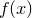# 11.35 list

```list
```

The list command returns a list of all of the items on the current multiplot canvas, giving their identification numbers and the commands used to produce them. The following is an example of the output produced:

pyxplot> list

```# ID   Command
1  plot item 1 f(x) using columns
2  [deleted] text item 2 "Figure 1: A plot of f(x)" at 0,0 rotate 0 gap 0
3  text item 3 "Figure 1: A plot of \$f(x)\$" at 0,0 rotate 0 gap 0
```

In this example, the user has plotted a graph ofand added a caption to it. The ID column lists the reference numbers of each multiplot item. Item 1 has been deleted.